# RS Aggarwal Solutions for Class 9 Chapter 19: Probability

The solutions are prepared in such a way it improves experimental approach among the students in solving problems. RS Aggarwal Solutions contain answers to all the exercise wise problems present in the textbook. These solutions are structured by mathematics experts having experience in the education industry. The experts prepare the solutions which are comprehensive in order to improve knowledge about the concepts among students.

The PDF of solutions can be used by the students to improve their confidence level and to attend the exams without fear. The concepts are explained in such a way that the students can remember the formulas and tips used to solve the problems quickly. RS Aggarwal Solutions for Class 9 Chapter 19 Probability are provided here.

## RS Aggarwal Solutions for Class 9 Chapter 19: Probability Download PDF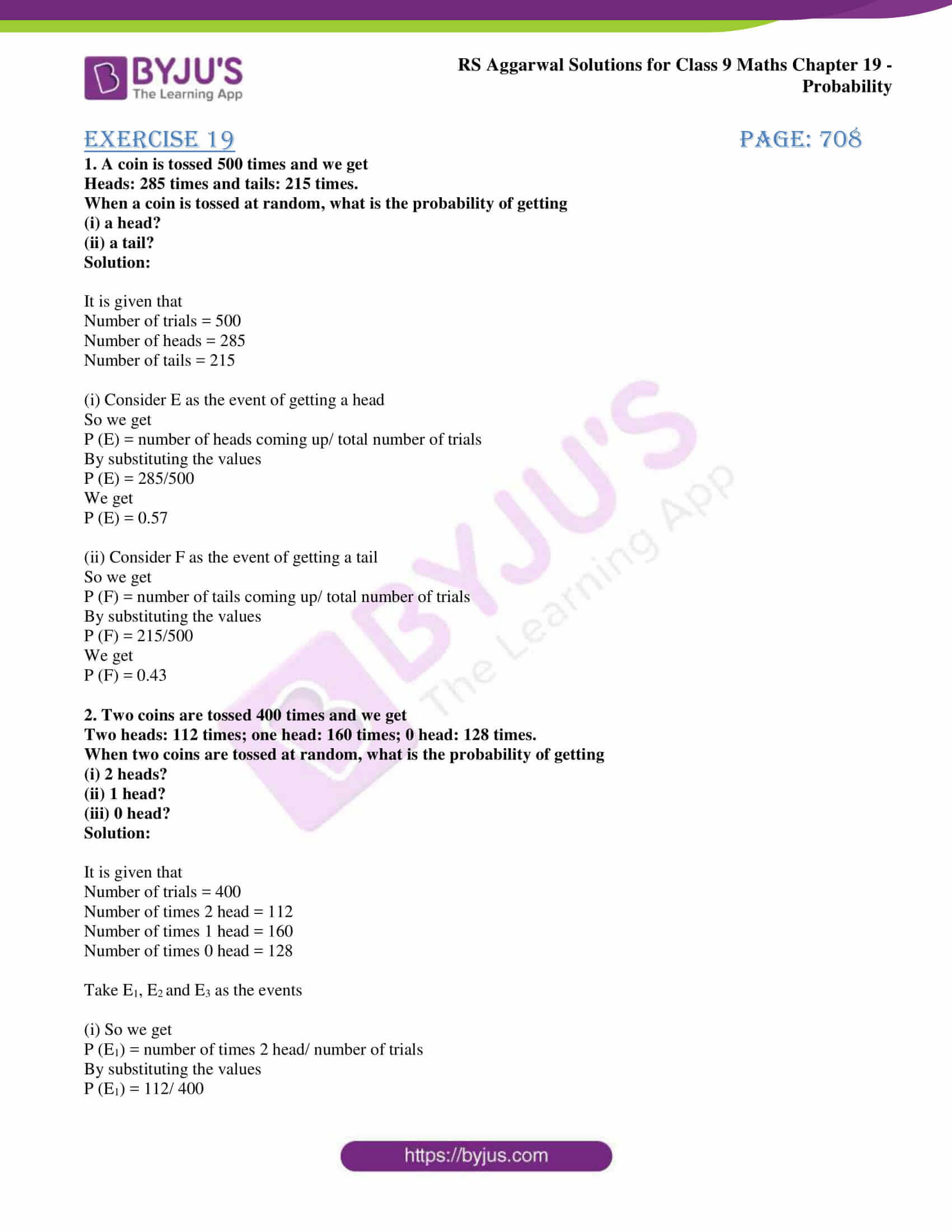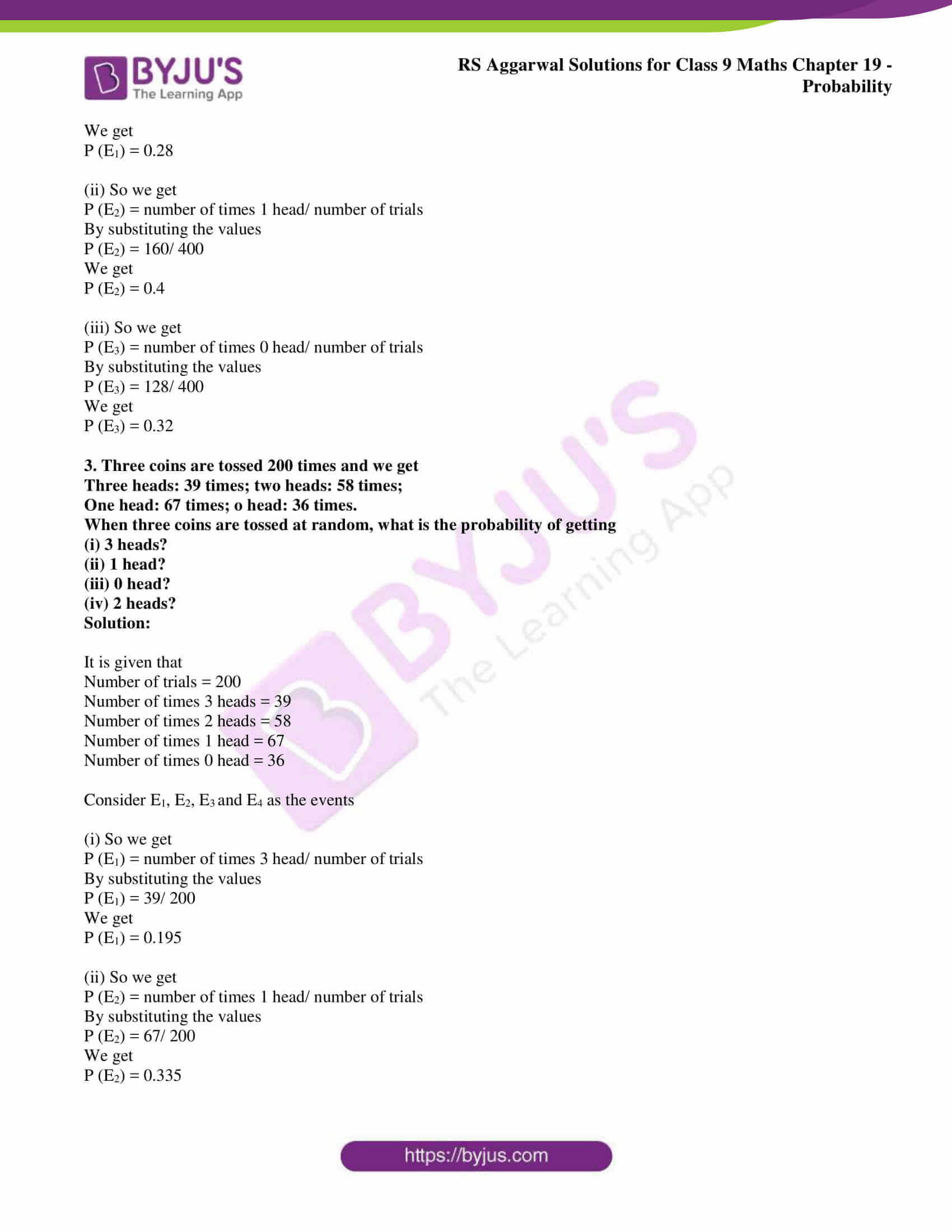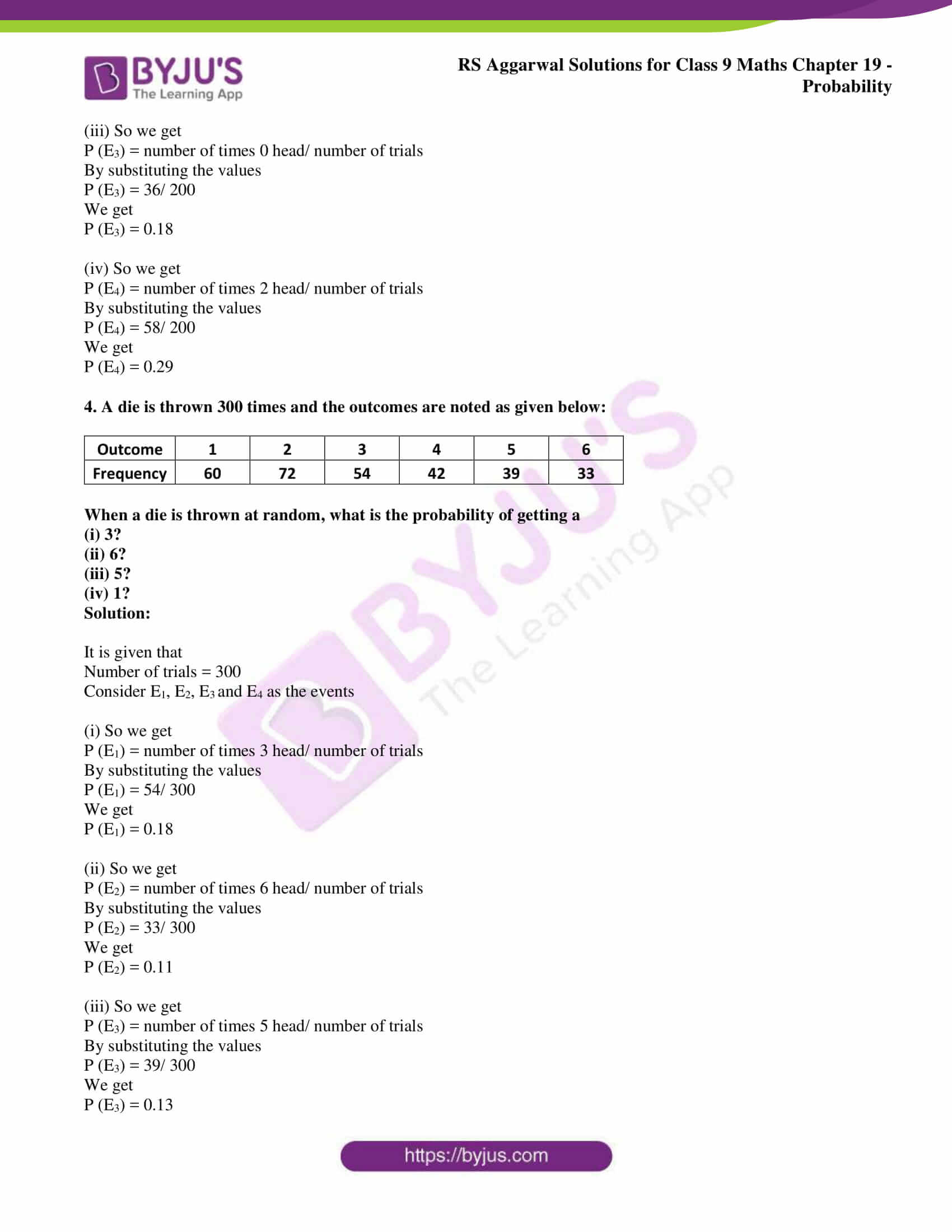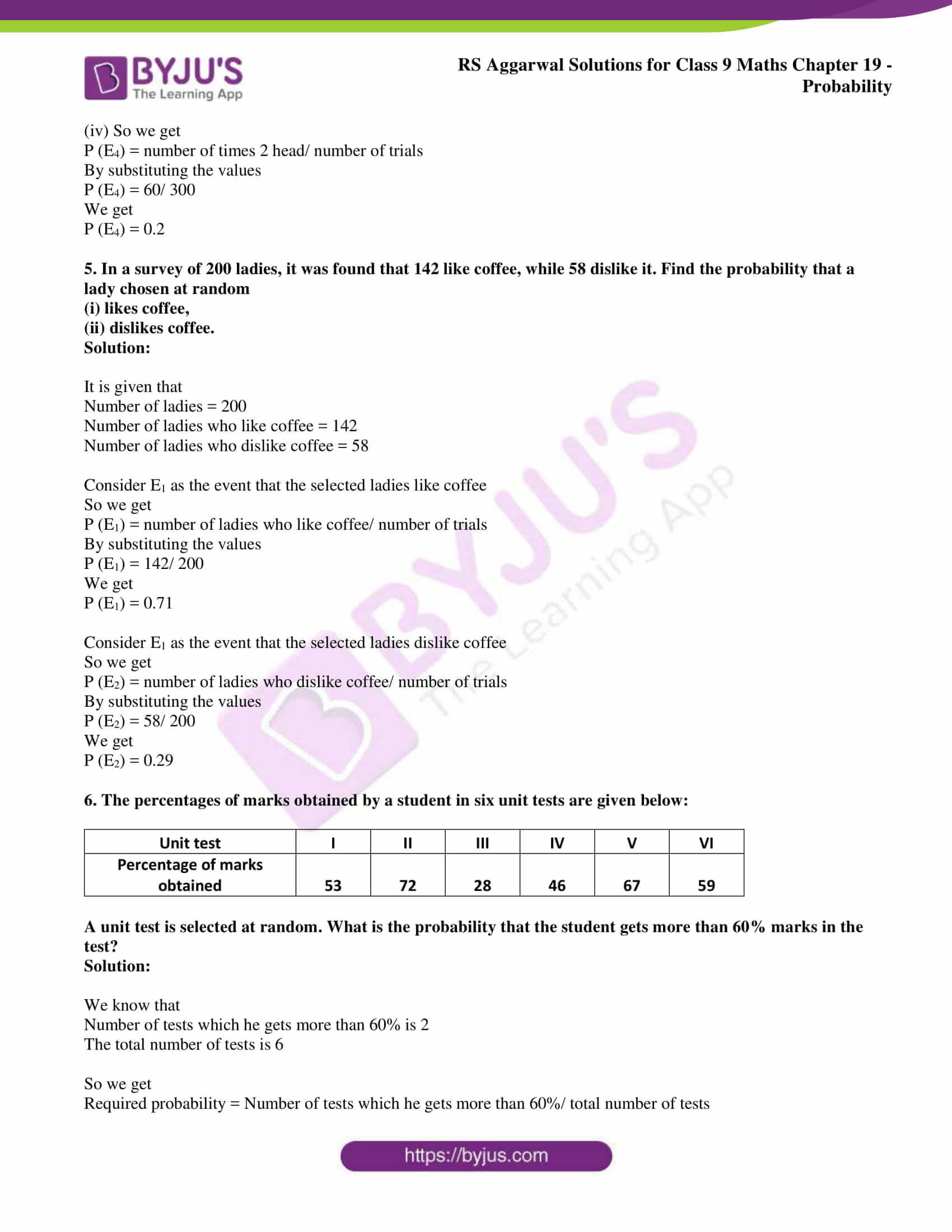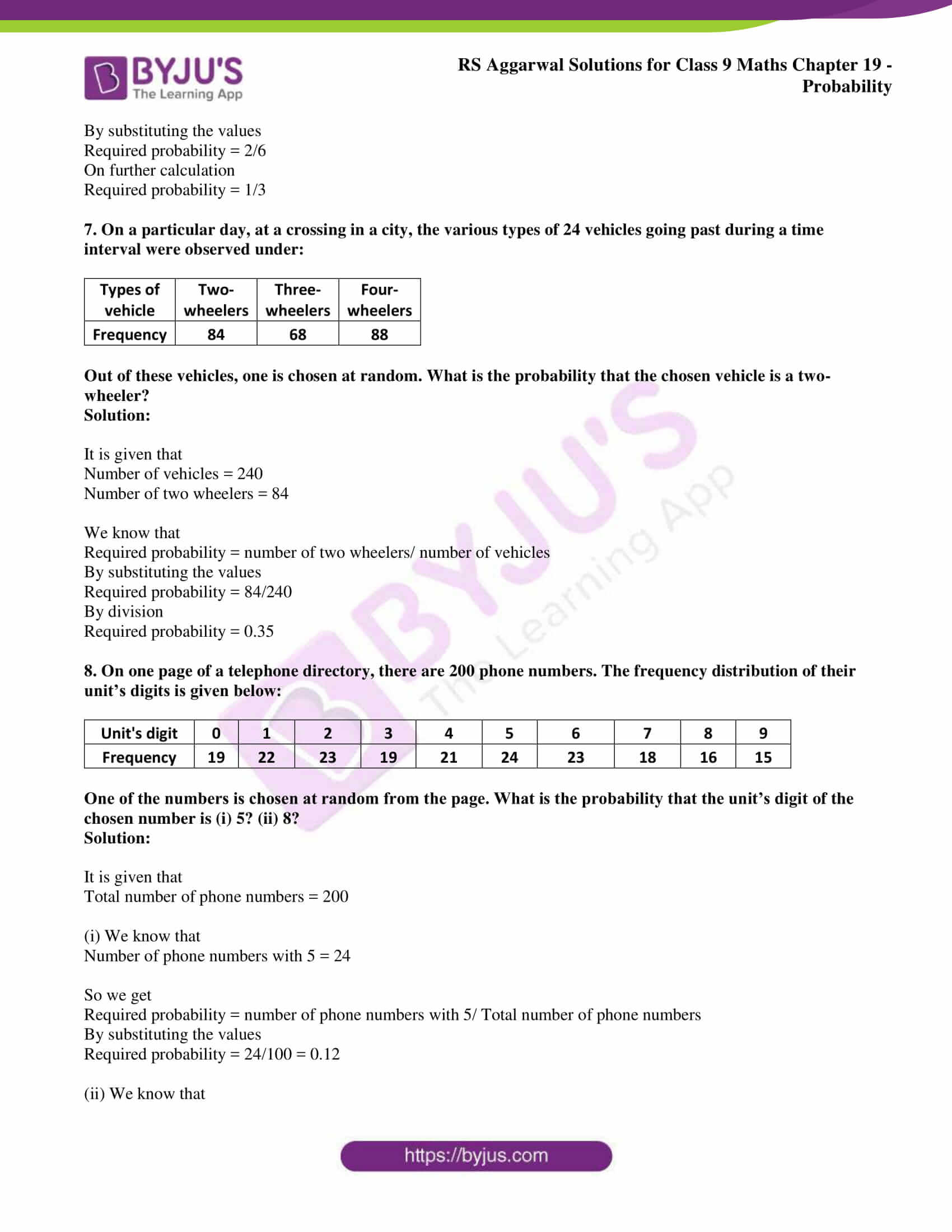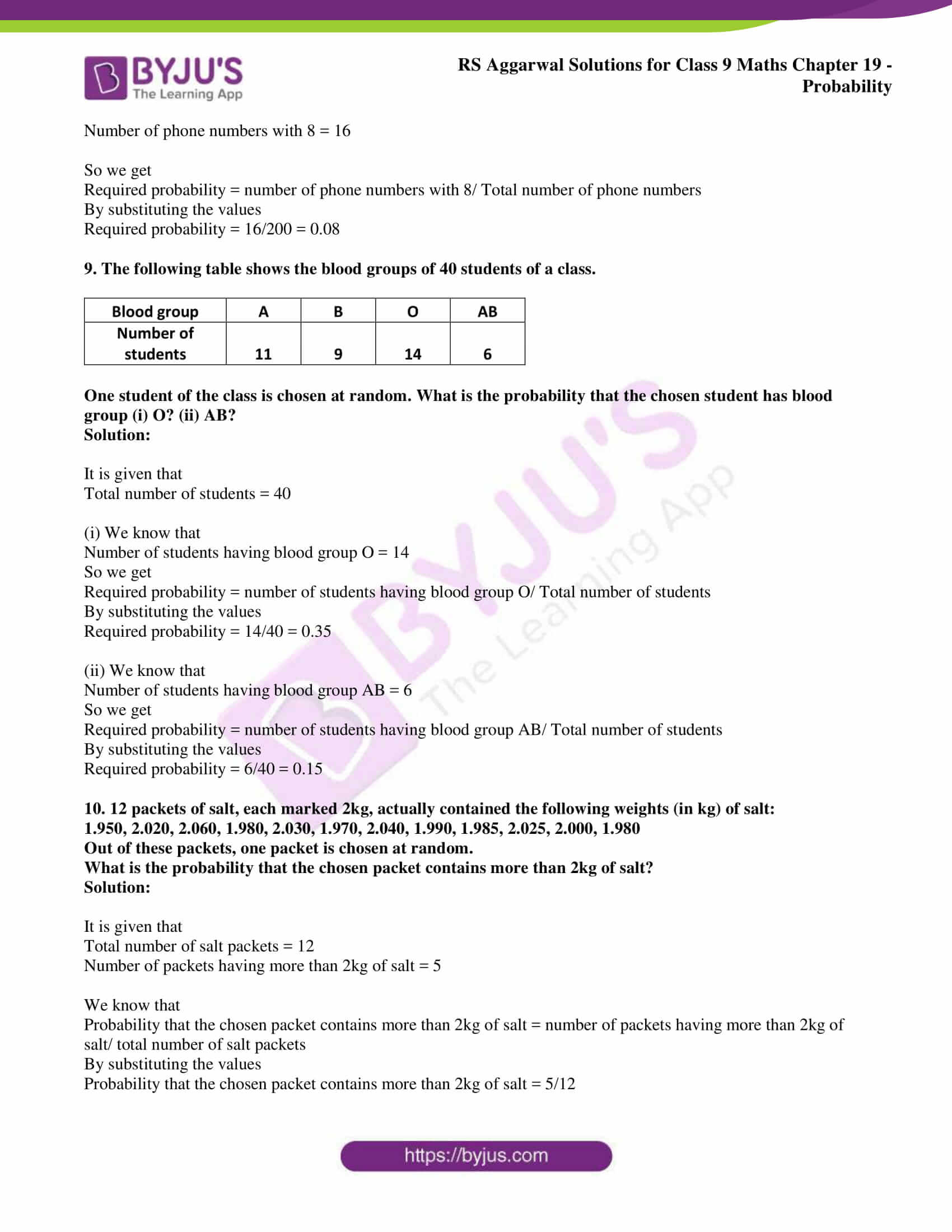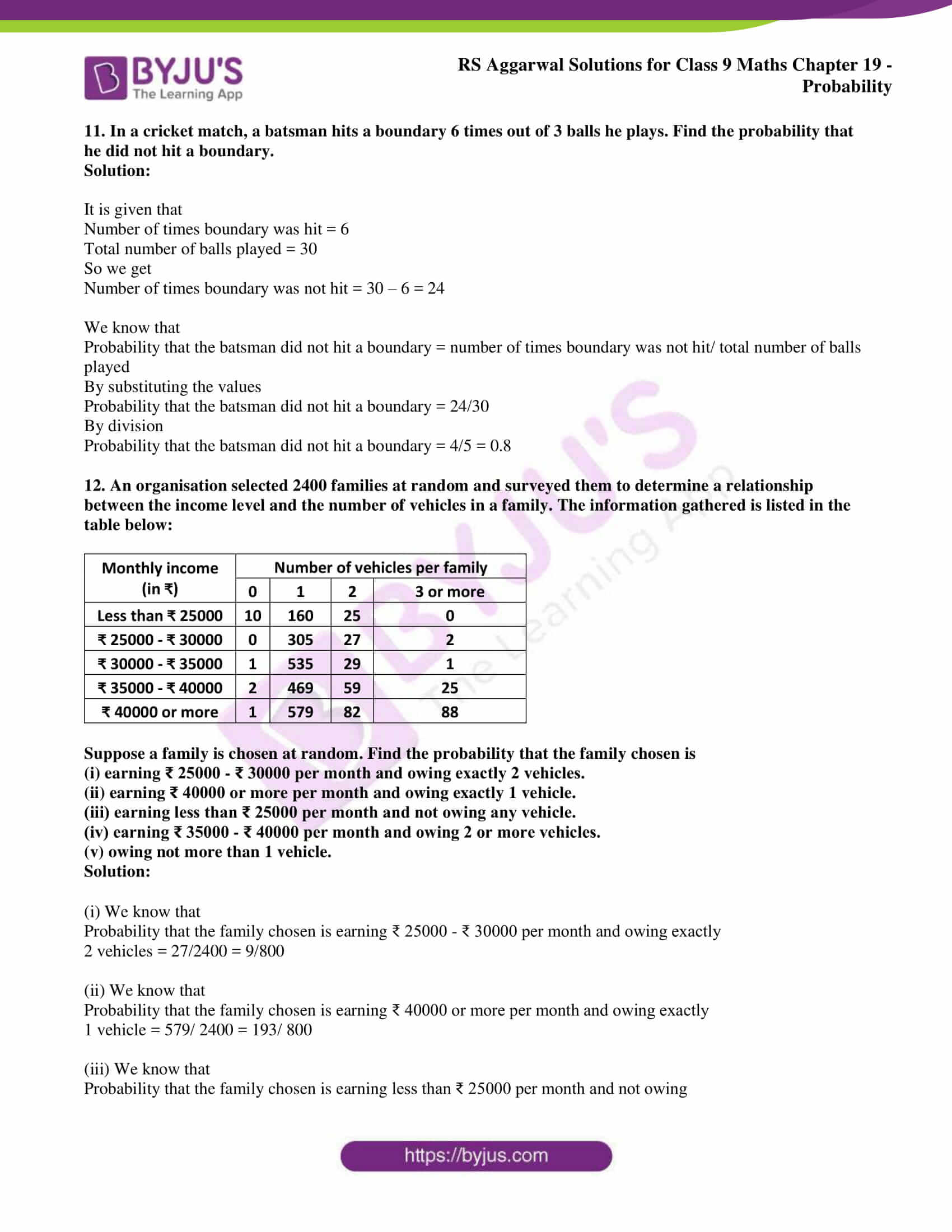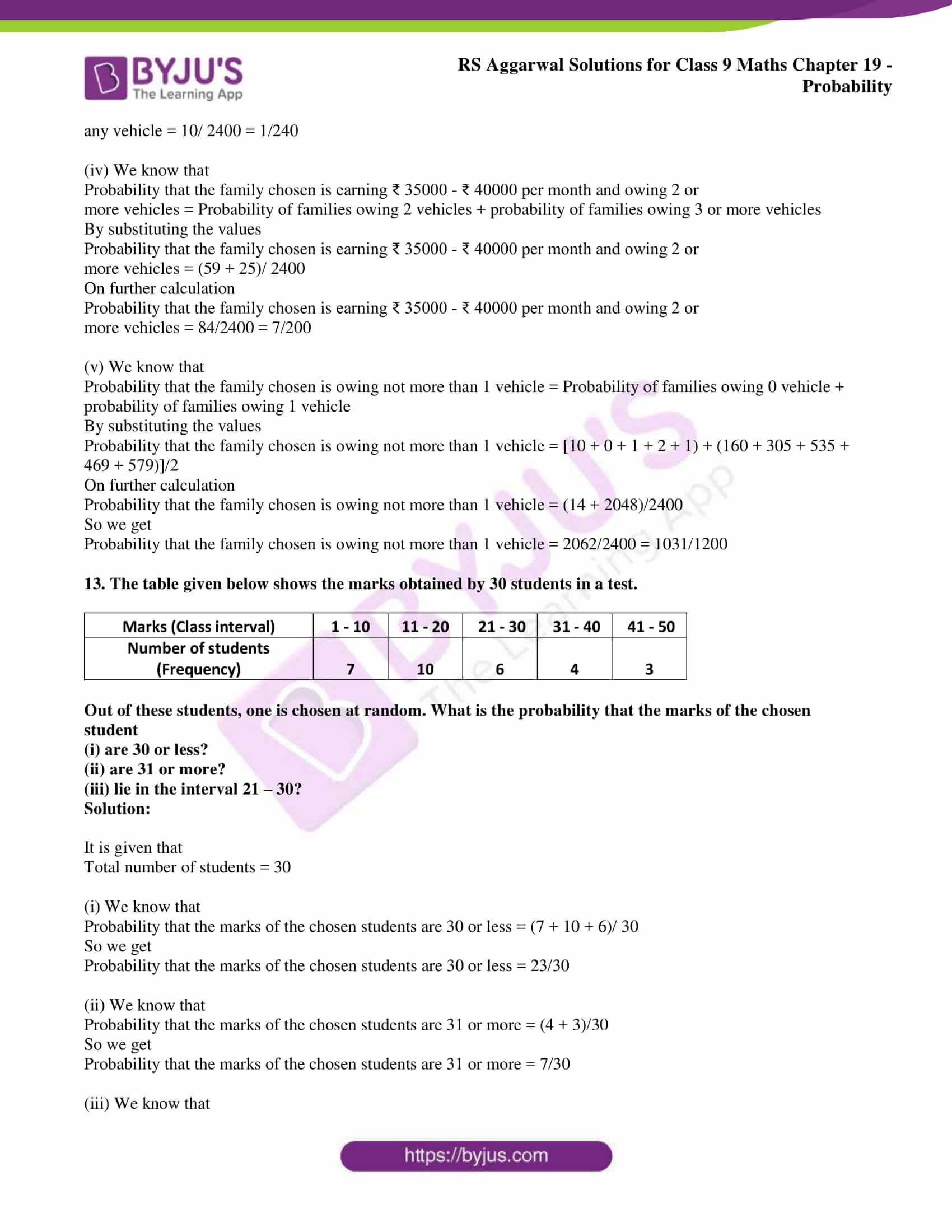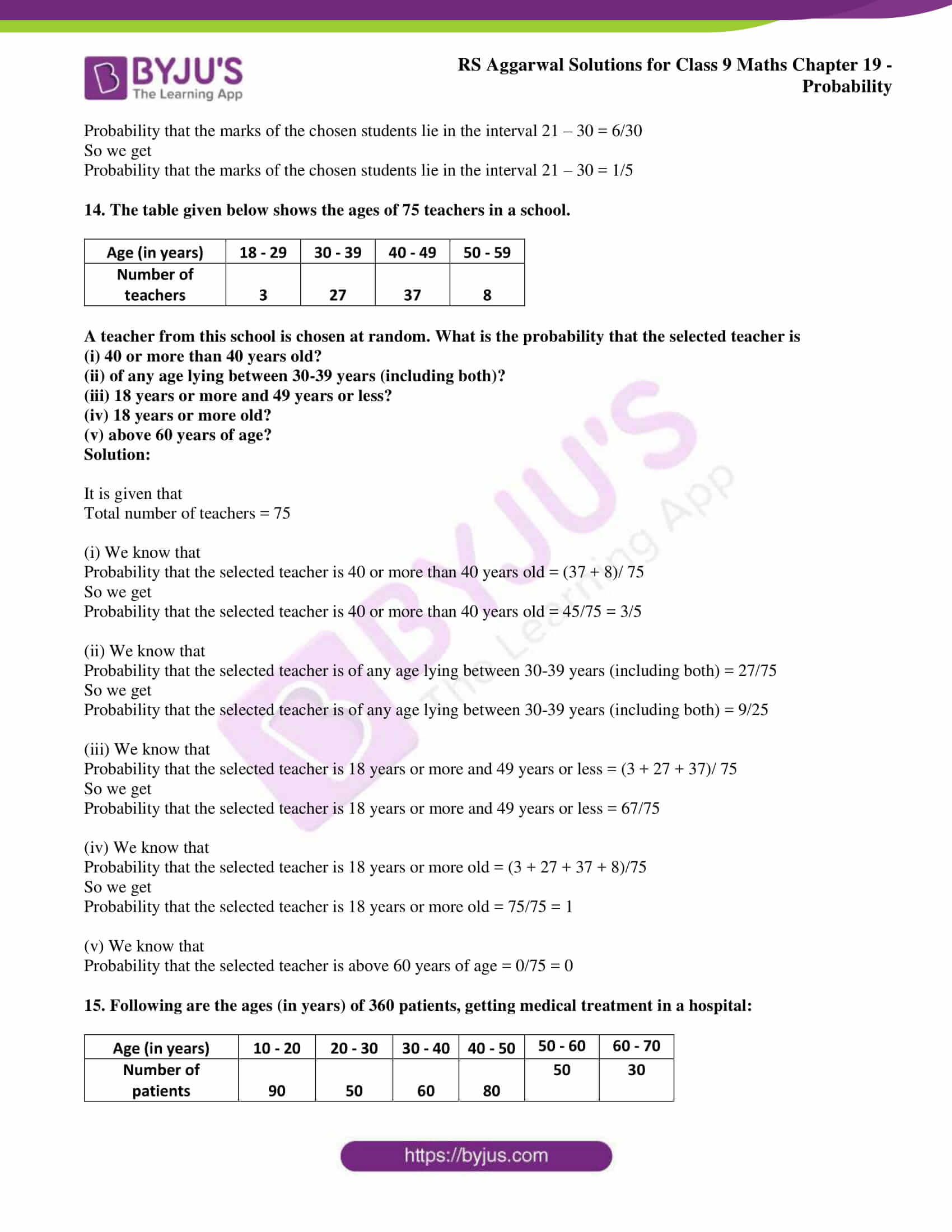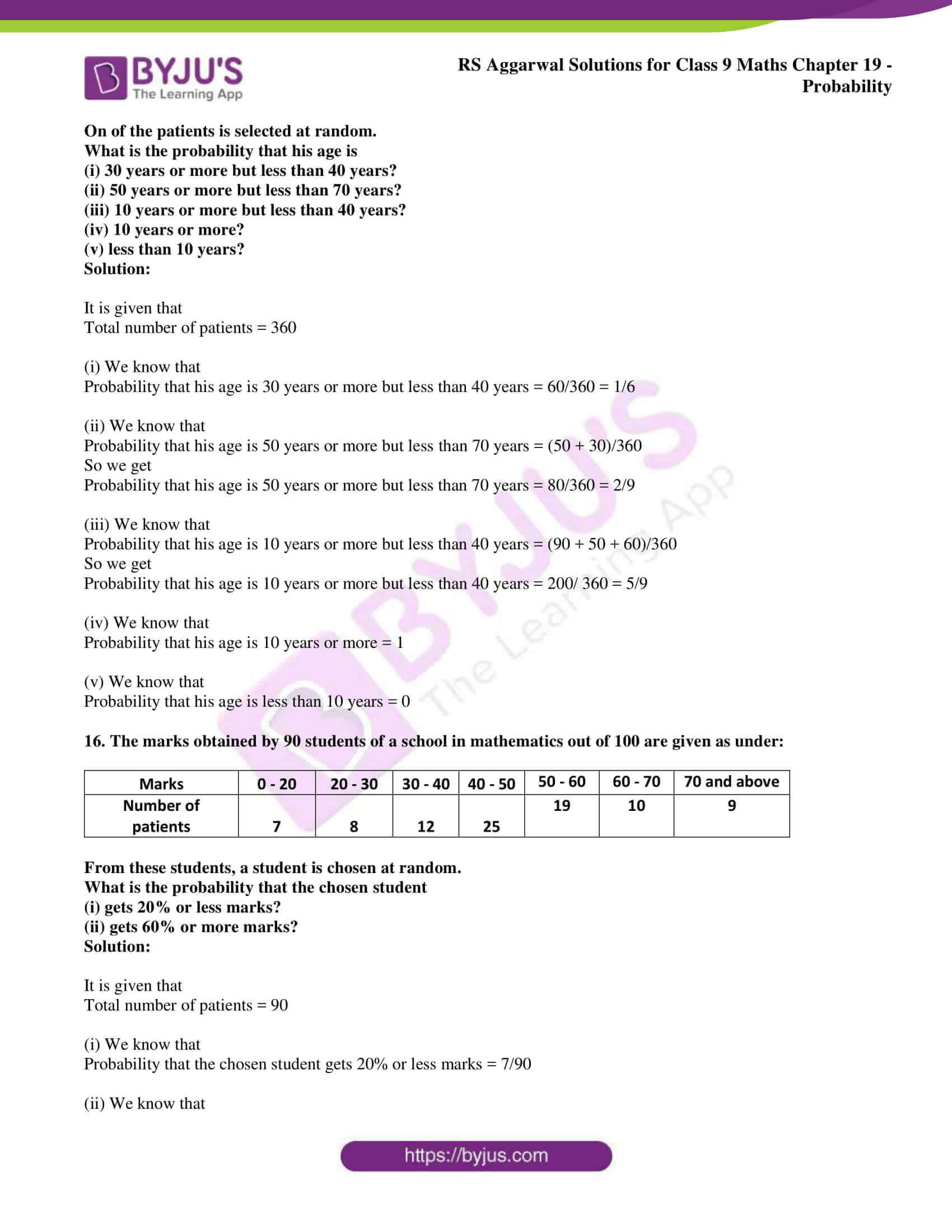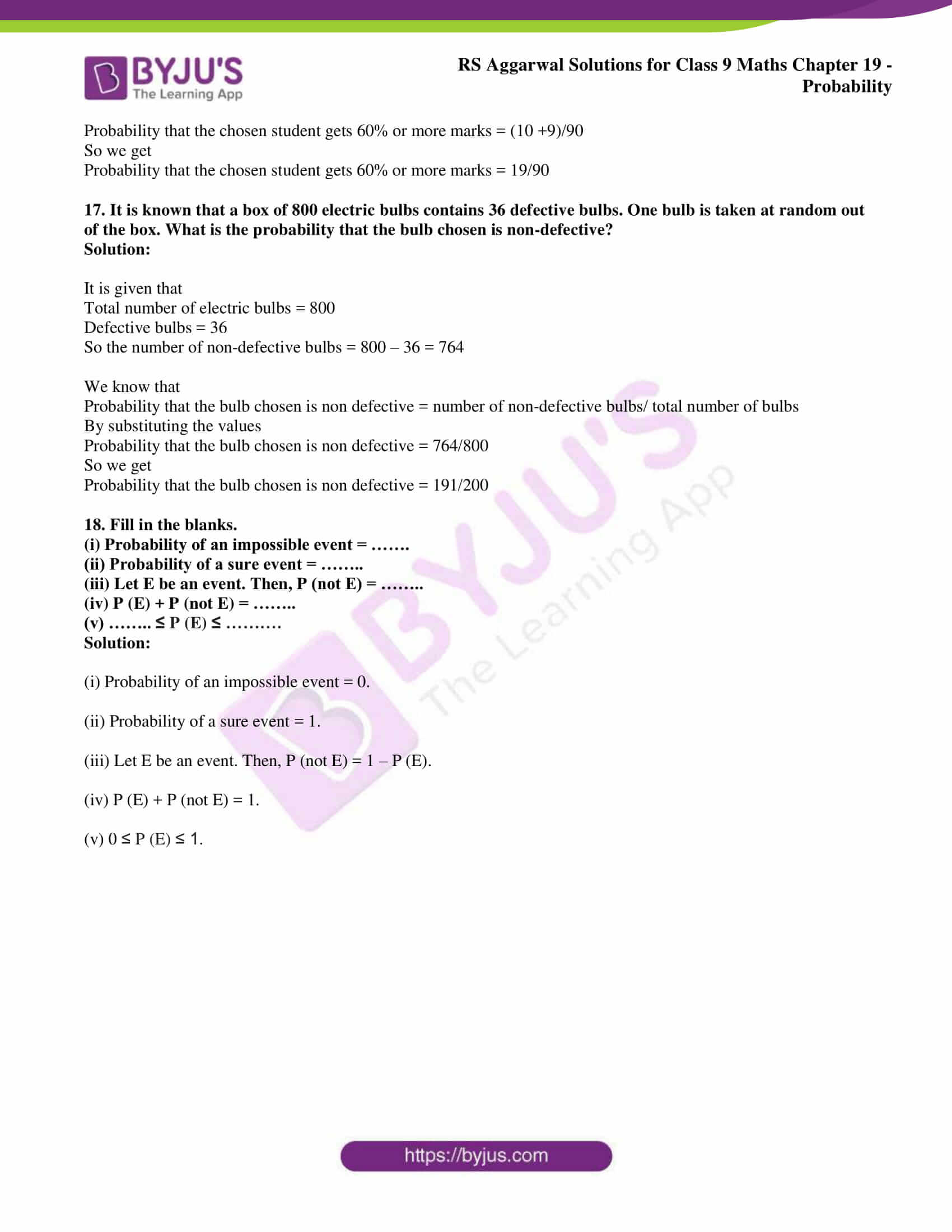## Access RS Aggarwal Solutions for Class 9 Chapter 19: Probability

Exercise 19 page: 708

1. A coin is tossed 500 times and we get

Heads: 285 times and tails: 215 times.

When a coin is tossed at random, what is the probability of getting

(ii) a tail?

Solution:

It is given that

Number of trials = 500

Number of tails = 215

(i) Consider E as the event of getting a head

So we get

P (E) = number of heads coming up/ total number of trials

By substituting the values

P (E) = 285/500

We get

P (E) = 0.57

(ii) Consider F as the event of getting a tail

So we get

P (F) = number of tails coming up/ total number of trials

By substituting the values

P (F) = 215/500

We get

P (F) = 0.43

2. Two coins are tossed 400 times and we get

When two coins are tossed at random, what is the probability of getting

Solution:

It is given that

Number of trials = 400

Number of times 2 head = 112

Number of times 1 head = 160

Number of times 0 head = 128

Take E1, E2 and E3 as the events

(i) So we get

P (E1) = number of times 2 head/ number of trials

By substituting the values

P (E1) = 112/ 400

We get

P (E1) = 0.28

(ii) So we get

P (E2) = number of times 1 head/ number of trials

By substituting the values

P (E2) = 160/ 400

We get

P (E2) = 0.4

(iii) So we get

P (E3) = number of times 0 head/ number of trials

By substituting the values

P (E3) = 128/ 400

We get

P (E3) = 0.32

3. Three coins are tossed 200 times and we get

When three coins are tossed at random, what is the probability of getting

Solution:

It is given that

Number of trials = 200

Number of times 3 heads = 39

Number of times 2 heads = 58

Number of times 1 head = 67

Number of times 0 head = 36

Consider E1, E2, E3 and E4 as the events

(i) So we get

P (E1) = number of times 3 head/ number of trials

By substituting the values

P (E1) = 39/ 200

We get

P (E1) = 0.195

(ii) So we get

P (E2) = number of times 1 head/ number of trials

By substituting the values

P (E2) = 67/ 200

We get

P (E2) = 0.335

(iii) So we get

P (E3) = number of times 0 head/ number of trials

By substituting the values

P (E3) = 36/ 200

We get

P (E3) = 0.18

(iv) So we get

P (E4) = number of times 2 head/ number of trials

By substituting the values

P (E4) = 58/ 200

We get

P (E4) = 0.29

4. A die is thrown 300 times and the outcomes are noted as given below:

 Outcome 1 2 3 4 5 6 Frequency 60 72 54 42 39 33

When a die is thrown at random, what is the probability of getting a

(i) 3?

(ii) 6?

(iii) 5?

(iv) 1?

Solution:

It is given that

Number of trials = 300

Consider E1, E2, E3 and E4 as the events

(i) So we get

P (E1) = number of times 3 head/ number of trials

By substituting the values

P (E1) = 54/ 300

We get

P (E1) = 0.18

(ii) So we get

P (E2) = number of times 6 head/ number of trials

By substituting the values

P (E2) = 33/ 300

We get

P (E2) = 0.11

(iii) So we get

P (E3) = number of times 5 head/ number of trials

By substituting the values

P (E3) = 39/ 300

We get

P (E3) = 0.13

(iv) So we get

P (E4) = number of times 2 head/ number of trials

By substituting the values

P (E4) = 60/ 300

We get

P (E4) = 0.2

5. In a survey of 200 ladies, it was found that 142 like coffee, while 58 dislike it. Find the probability that a lady chosen at random

(i) likes coffee,

(ii) dislikes coffee.

Solution:

It is given that

Number of ladies who like coffee = 142

Number of ladies who dislike coffee = 58

Consider E1 as the event that the selected ladies like coffee

So we get

P (E1) = number of ladies who like coffee/ number of trials

By substituting the values

P (E1) = 142/ 200

We get

P (E1) = 0.71

Consider E1 as the event that the selected ladies dislike coffee

So we get

P (E2) = number of ladies who dislike coffee/ number of trials

By substituting the values

P (E2) = 58/ 200

We get

P (E2) = 0.29

6. The percentages of marks obtained by a student in six unit tests are given below:

 Unit test I II III IV V VI Percentage of marks obtained 53 72 28 46 67 59

A unit test is selected at random. What is the probability that the student gets more than 60% marks in the test?

Solution:

We know that

Number of tests which he gets more than 60% is 2

The total number of tests is 6

So we get

Required probability = Number of tests which he gets more than 60%/ total number of tests

By substituting the values

Required probability = 2/6

On further calculation

Required probability = 1/3

7. On a particular day, at a crossing in a city, the various types of 24 vehicles going past during a time interval were observed under:

 Types of vehicle Two-wheelers Three-wheelers Four-wheelers Frequency 84 68 88

Out of these vehicles, one is chosen at random. What is the probability that the chosen vehicle is a two-wheeler?

Solution:

It is given that

Number of vehicles = 240

Number of two wheelers = 84

We know that

Required probability = number of two wheelers/ number of vehicles

By substituting the values

Required probability = 84/240

By division

Required probability = 0.35

8. On one page of a telephone directory, there are 200 phone numbers. The frequency distribution of their unit’s digits is given below:

 Unit’s digit 0 1 2 3 4 5 6 7 8 9 Frequency 19 22 23 19 21 24 23 18 16 15

One of the numbers is chosen at random from the page. What is the probability that the unit’s digit of the chosen number is (i) 5? (ii) 8?

Solution:

It is given that

Total number of phone numbers = 200

(i) We know that

Number of phone numbers with 5 = 24

So we get

Required probability = number of phone numbers with 5/ Total number of phone numbers

By substituting the values

Required probability = 24/100 = 0.12

(ii) We know that

Number of phone numbers with 8 = 16

So we get

Required probability = number of phone numbers with 8/ Total number of phone numbers

By substituting the values

Required probability = 16/200 = 0.08

9. The following table shows the blood groups of 40 students of a class.

 Blood group A B O AB Number of students 11 9 14 6

One student of the class is chosen at random. What is the probability that the chosen student has blood group (i) O? (ii) AB?

Solution:

It is given that

Total number of students = 40

(i) We know that

Number of students having blood group O = 14

So we get

Required probability = number of students having blood group O/ Total number of students

By substituting the values

Required probability = 14/40 = 0.35

(ii) We know that

Number of students having blood group AB = 6

So we get

Required probability = number of students having blood group AB/ Total number of students

By substituting the values

Required probability = 6/40 = 0.15

10. 12 packets of salt, each marked 2kg, actually contained the following weights (in kg) of salt:

1.950, 2.020, 2.060, 1.980, 2.030, 1.970, 2.040, 1.990, 1.985, 2.025, 2.000, 1.980

Out of these packets, one packet is chosen at random.

What is the probability that the chosen packet contains more than 2kg of salt?

Solution:

It is given that

Total number of salt packets = 12

Number of packets having more than 2kg of salt = 5

We know that

Probability that the chosen packet contains more than 2kg of salt = number of packets having more than 2kg of salt/ total number of salt packets

By substituting the values

Probability that the chosen packet contains more than 2kg of salt = 5/12

11. In a cricket match, a batsman hits a boundary 6 times out of 3 balls he plays. Find the probability that he did not hit a boundary.

Solution:

It is given that

Number of times boundary was hit = 6

Total number of balls played = 30

So we get

Number of times boundary was not hit = 30 – 6 = 24

We know that

Probability that the batsman did not hit a boundary = number of times boundary was not hit/ total number of balls played

By substituting the values

Probability that the batsman did not hit a boundary = 24/30

By division

Probability that the batsman did not hit a boundary = 4/5 = 0.8

12. An organisation selected 2400 families at random and surveyed them to determine a relationship between the income level and the number of vehicles in a family. The information gathered is listed in the table below:

 Monthly income (in ₹) Number of vehicles per family 0 1 2 3 or more Less than ₹ 25000 10 160 25 0 ₹ 25000 – ₹ 30000 0 305 27 2 ₹ 30000 – ₹ 35000 1 535 29 1 ₹ 35000 – ₹ 40000 2 469 59 25 ₹ 40000 or more 1 579 82 88

Suppose a family is chosen at random. Find the probability that the family chosen is

(i) earning ₹ 25000 – ₹ 30000 per month and owing exactly 2 vehicles.

(ii) earning ₹ 40000 or more per month and owing exactly 1 vehicle.

(iii) earning less than ₹ 25000 per month and not owing any vehicle.

(iv) earning ₹ 35000 – ₹ 40000 per month and owing 2 or more vehicles.

(v) owing not more than 1 vehicle.

Solution:

(i) We know that

Probability that the family chosen is earning ₹ 25000 – ₹ 30000 per month and owing exactly

2 vehicles = 27/2400 = 9/800

(ii) We know that

Probability that the family chosen is earning ₹ 40000 or more per month and owing exactly

1 vehicle = 579/ 2400 = 193/ 800

(iii) We know that

Probability that the family chosen is earning less than ₹ 25000 per month and not owing

any vehicle = 10/ 2400 = 1/240

(iv) We know that

Probability that the family chosen is earning ₹ 35000 – ₹ 40000 per month and owing 2 or

more vehicles = Probability of families owing 2 vehicles + probability of families owing 3 or more vehicles

By substituting the values

Probability that the family chosen is earning ₹ 35000 – ₹ 40000 per month and owing 2 or

more vehicles = (59 + 25)/ 2400

On further calculation

Probability that the family chosen is earning ₹ 35000 – ₹ 40000 per month and owing 2 or

more vehicles = 84/2400 = 7/200

(v) We know that

Probability that the family chosen is owing not more than 1 vehicle = Probability of families owing 0 vehicle + probability of families owing 1 vehicle

By substituting the values

Probability that the family chosen is owing not more than 1 vehicle = [10 + 0 + 1 + 2 + 1) + (160 + 305 + 535 + 469 + 579)]/2

On further calculation

Probability that the family chosen is owing not more than 1 vehicle = (14 + 2048)/2400

So we get

Probability that the family chosen is owing not more than 1 vehicle = 2062/2400 = 1031/1200

13. The table given below shows the marks obtained by 30 students in a test.

 Marks (Class interval) 1 – 10 11 – 20 21 – 30 31 – 40 41 – 50 Number of students (Frequency) 7 10 6 4 3

Out of these students, one is chosen at random. What is the probability that the marks of the chosen student

(i) are 30 or less?

(ii) are 31 or more?

(iii) lie in the interval 21 – 30?

Solution:

It is given that

Total number of students = 30

(i) We know that

Probability that the marks of the chosen students are 30 or less = (7 + 10 + 6)/ 30

So we get

Probability that the marks of the chosen students are 30 or less = 23/30

(ii) We know that

Probability that the marks of the chosen students are 31 or more = (4 + 3)/30

So we get

Probability that the marks of the chosen students are 31 or more = 7/30

(iii) We know that

Probability that the marks of the chosen students lie in the interval 21 – 30 = 6/30

So we get

Probability that the marks of the chosen students lie in the interval 21 – 30 = 1/5

14. The table given below shows the ages of 75 teachers in a school.

 Age (in years) 18 – 29 30 – 39 40 – 49 50 – 59 Number of teachers 3 27 37 8

A teacher from this school is chosen at random. What is the probability that the selected teacher is

(i) 40 or more than 40 years old?

(ii) of any age lying between 30-39 years (including both)?

(iii) 18 years or more and 49 years or less?

(iv) 18 years or more old?

(v) above 60 years of age?

Solution:

It is given that

Total number of teachers = 75

(i) We know that

Probability that the selected teacher is 40 or more than 40 years old = (37 + 8)/ 75

So we get

Probability that the selected teacher is 40 or more than 40 years old = 45/75 = 3/5

(ii) We know that

Probability that the selected teacher is of any age lying between 30-39 years (including both) = 27/75

So we get

Probability that the selected teacher is of any age lying between 30-39 years (including both) = 9/25

(iii) We know that

Probability that the selected teacher is 18 years or more and 49 years or less = (3 + 27 + 37)/ 75

So we get

Probability that the selected teacher is 18 years or more and 49 years or less = 67/75

(iv) We know that

Probability that the selected teacher is 18 years or more old = (3 + 27 + 37 + 8)/75

So we get

Probability that the selected teacher is 18 years or more old = 75/75 = 1

(v) We know that

Probability that the selected teacher is above 60 years of age = 0/75 = 0

15. Following are the ages (in years) of 360 patients, getting medical treatment in a hospital:

 Age (in years) 10 – 20 20 – 30 30 – 40 40 – 50 50 – 60 60 – 70 Number of patients 90 50 60 80 50 30

On of the patients is selected at random.

What is the probability that his age is

(i) 30 years or more but less than 40 years?

(ii) 50 years or more but less than 70 years?

(iii) 10 years or more but less than 40 years?

(iv) 10 years or more?

(v) less than 10 years?

Solution:

It is given that

Total number of patients = 360

(i) We know that

Probability that his age is 30 years or more but less than 40 years = 60/360 = 1/6

(ii) We know that

Probability that his age is 50 years or more but less than 70 years = (50 + 30)/360

So we get

Probability that his age is 50 years or more but less than 70 years = 80/360 = 2/9

(iii) We know that

Probability that his age is 10 years or more but less than 40 years = (90 + 50 + 60)/360

So we get

Probability that his age is 10 years or more but less than 40 years = 200/ 360 = 5/9

(iv) We know that

Probability that his age is 10 years or more = 1

(v) We know that

Probability that his age is less than 10 years = 0

16. The marks obtained by 90 students of a school in mathematics out of 100 are given as under:

 Marks 0 – 20 20 – 30 30 – 40 40 – 50 50 – 60 60 – 70 70 and above Number of patients 7 8 12 25 19 10 9

From these students, a student is chosen at random.

What is the probability that the chosen student

(i) gets 20% or less marks?

(ii) gets 60% or more marks?

Solution:

It is given that

Total number of patients = 90

(i) We know that

Probability that the chosen student gets 20% or less marks = 7/90

(ii) We know that

Probability that the chosen student gets 60% or more marks = (10 +9)/90

So we get

Probability that the chosen student gets 60% or more marks = 19/90

17. It is known that a box of 800 electric bulbs contains 36 defective bulbs. One bulb is taken at random out of the box. What is the probability that the bulb chosen is non-defective?

Solution:

It is given that

Total number of electric bulbs = 800

Defective bulbs = 36

So the number of non-defective bulbs = 800 – 36 = 764

We know that

Probability that the bulb chosen is non defective = number of non-defective bulbs/ total number of bulbs

By substituting the values

Probability that the bulb chosen is non defective = 764/800

So we get

Probability that the bulb chosen is non defective = 191/200

18. Fill in the blanks.

(i) Probability of an impossible event = …….

(ii) Probability of a sure event = ……..

(iii) Let E be an event. Then, P (not E) = ……..

(iv) P (E) + P (not E) = ……..

(v) …….. ≤ P (E) ≤ ……….

Solution:

(i) Probability of an impossible event = 0.

(ii) Probability of a sure event = 1.

(iii) Let E be an event. Then, P (not E) = 1 – P (E).

(iv) P (E) + P (not E) = 1.

(v) 0 ≤ P (E) ≤ 1.

### RS Aggarwal Solutions for Class 9 Maths Chapter 19: Probability

Chapter 19, Probability, has 1 exercise which has problems solved based on the concepts which are covered in the chapter. The topics of greater importance which are discussed in RS Aggarwal Solutions Chapter 19 are as follows:

• Introduction
• History
• Some terms related to probability
• Some operations and their outcomes

### RS Aggarwal Solutions Class 9 Maths Chapter 19 – Probability

RS Aggarwal Solutions which are provided in PDF format are simple, authentic and easy to understand. These solutions contain answers to the exercise wise questions based on the RS Aggarwal textbook for Class 9. The main aim is to provide students with different methods which can be used to solve problems faster. The solutions are prepared by subject experts after huge research on the topics.

The students can rely on PDF of the solutions which help in grasping the formulas, shortcuts and tricks to solve the problems according to the CBSE exam pattern. Some of the major applications of Probability are sports strategies, forecasts, games and recreational activities, insurance options and in business.